C Program to Count number of digits in number without using mod operator

In this tutorial you will learn about the C Program to Count number of digits in number without using mod operator and its application with practical example.

C Program to Count the number of digits in number without using the mod operator.

In this tutorial, we will learn to create a C program that will Count the number of digits in number without using the mod operator in C programming.

Prerequisites

Before starting with this tutorial we assume that you are best aware of the following C programming topics:

• Operators in C Programming.
• Basic Input and Output function in C Programming.
• Basic C programming.
• String functions in c programming.

Count the number of digits in number without using the mod operator.

As we all know the c programming is a very powerful language. In the c programming language, we have many pre-defined functions for arithmetic operations. but today we will count the number of digits in a number without using the mod operator.

With the help of this program, we can count the number of digits in numbers without using the mod operator.

Output:-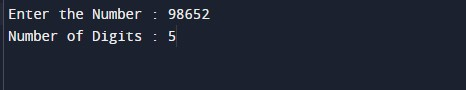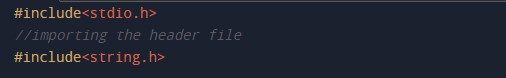In the above program, we have first initialized the required variable.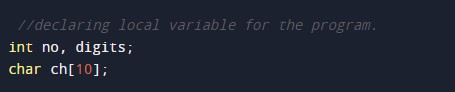• ch = it will hold the string value.
• digits = it will hold the integer value for numbers.
• no = it will hold the integer value of number input.

Taking input number from the user.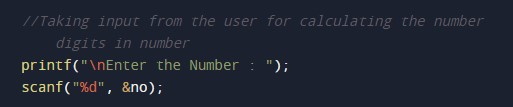Converting that number to a string.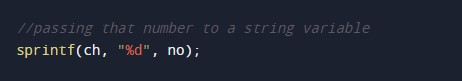Storing the string size by using a predefined function.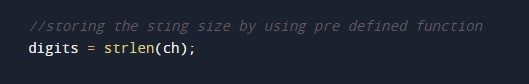Printing output of the program.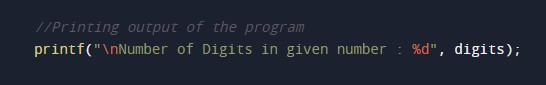In this tutorial we have learn about the C Program to Count number of digits in number without using mod operator and its application with practical example. I hope you will like this tutorial.